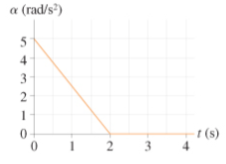# Problem: The figure(Figure 1) shows the angular acceleration graph of a turntable that starts from rest.What is the turntable's angular velocity at t = 2.0s? Express your answer to two significant figures and include the appropriate units.

###### FREE Expert Solution

Angular acceleration is given by:

$\overline{){\mathbf{\alpha }}{\mathbf{=}}\frac{\mathbf{d}\mathbf{\omega }}{\mathbf{d}\mathbf{t}}}$

Solving for angular velocity:

100% (490 ratings)###### Problem Details

The figure(Figure 1) shows the angular acceleration graph of a turntable that starts from rest.What is the turntable's angular velocity at t = 2.0s? Express your answer to two significant figures and include the appropriate units.